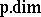# Tilted algebra

The endomorphism ring of a tilting module over a finite-dimensional hereditary algebra (cf. also Algebra; Endomorphism).

Let $H$ be a finite-dimensional hereditary $K$-algebra, $K$ some field, for example the path-algebra of some finite quiver without oriented cycles. A finite-dimensional $H$-module $\square _ { H } T$ is called a tilting module if

i) $\operatorname {p.dim} T \leq 1$, which always is satisfied in this context;

ii) $\operatorname { Ext } _ { H } ^ { 1 } ( T , T ) = 0$; and

iii) there exists a short exact sequence $0 \rightarrow H \rightarrow T _ { 1 } \rightarrow T _ { 2 } \rightarrow 0$ with $T _ { 1 }$ andin $\operatorname{add} T$, the category of finite direct sums of direct summands of $T$. Here,is projective dimension. The third condition also says that $T$ is maximal with respect to the property $\operatorname { Ext } _ { H } ^ { 1 } ( T , T ) = 0$. Note further, that a tilting module $T$ over a hereditary algebra is uniquely determined by its composition factors. Cf. also Tilting module.

The algebra $B = \operatorname { End } _ { H } ( T )$ is called a tilted algebra of type $H$, and $T$ becomes an $( H , B )$-bimodule (cf. also Bimodule).

In $H$-mod, the category of finite-dimensional $H$-modules, the module $T$ defines a torsion pair $( \mathcal{G} , \mathcal{F} )$ with torsion class $\mathcal{G}$ consisting of modules, generated by $T$ and torsion-free class $\mathcal{F} = \{ Y : \operatorname { Hom } _ { H } ( T , Y ) = 0 \}$. In $B$-mod it defines the torsion pair $\cal ( X , Y )$ with torsion class $\chi = \{ Y : T \otimes _ { B } Y = 0 \}$ and torsion-free class $\mathcal{Y} = \{ Y : \operatorname { Tor } _ { 1 } ^ { B } ( T , Y ) = 0 \}$. The Brenner–Butler theorem says that the functors $\operatorname{Hom}_H( T , - )$, respectively $T \otimes_{ B} -$, induce equivalences between $\mathcal{G}$ and $\mathcal{Y}$, whereas $\operatorname { Ext } _ { H } ^ { 1 } ( T , - )$, respectively $\operatorname { Tor } _ { 1 } ^ { B } ( T , - )$, induce equivalences between $\mathcal{F}$ and $\mathcal{X}$. In $B$-mod the torsion pair is splitting, that is, any indecomposable $B$-module is either torsion or torsion-free. In this sense, $B$-mod has "less" indecomposable modules, and information on the category $H$-mod can be transferred to $B$-mod.

For example, $B$ has global dimension at most $2$ and any indecomposable $B$-module has projective dimension or injective dimension at most $1$ (cf. also Dimension for dimension notions). These condition characterize the more general class of quasi-tilted algebras.

The indecomposable injective $H$-modules are in the torsion class $\mathcal{G}$ and their images under the tilting functor $\operatorname{Hom}_H( T , - )$ are contained in one connected component of the Auslander–Reiten quiver $\Gamma ( B )$ of $B$-mod (cf. also Quiver; Riedtmann classification), and they form a complete slice in this component. Moreover, the existence of such a complete slice in a connected component of $\Gamma ( B )$ characterizes tilted algebras. Moreover, the Auslander–Reiten quiver of a tilted algebra always contains pre-projective and pre-injective components.

If $H$ is a basic hereditary algebra and $H e$ is a simple projective module, then $T = H ( 1 - e ) \oplus \operatorname { TrD } H e$, where $\operatorname{Tr}D$ denotes the Auslander–Reiten translation (cf. Riedtmann classification), is a tilting module, sometimes called APR-tilting module. The induced torsion pair $( \mathcal{G} , \mathcal{F} )$ in $H$-mod is splitting and $H e$ is the unique indecomposable module in $\mathcal{F}$. The tilting functor $\operatorname{Hom}_H( T , - )$ corresponds to the reflection functor introduced by I.N. Bernshtein, I.M. Gel'fand and V.A. Ponomarev for their proof of the Gabriel theorem [a4].

If the hereditary algebra $H$ is representation-finite (cf. also Algebra of finite representation type), then any tilted algebra of type $H$ also is representation-finite. If $H$ is tame (cf. also Representation of an associative algebra), then a tilted algebra of type $H$ either is tame and one-parametric, or representation-finite. The latter case is equivalent to the fact that the tilting module contains non-zero pre-projective and pre-injective direct summands simultaneously. If $H$ is wild (cf. also Representation of an associative algebra), then a tilted algebra of type $H$ may be wild, or tame domestic, or representation-finite.